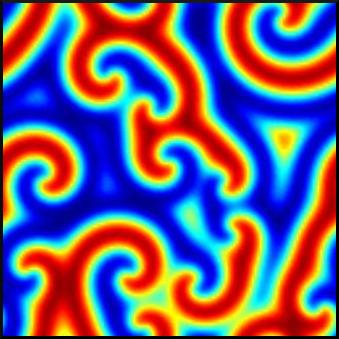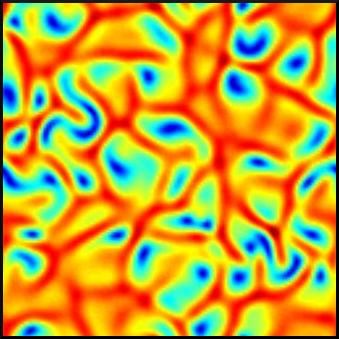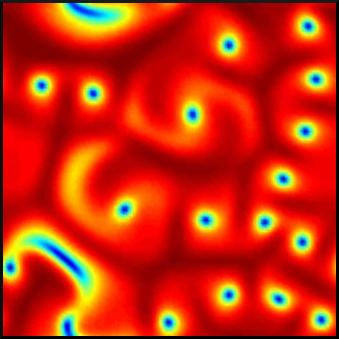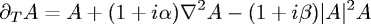# The Complex Ginzburg-Landau equation

 Applied Mathematics School of Mathematical Sciences University of Nottingham Nottingham NG7 2RD, UK david.winterbottom@gmail.comAbstract

The complex Ginzburg-Landau equation:is one of the most-studied equations in applied mathematics. It describes qualitatively, and often quantitatively, a vast array of phenomena including nonlinear waves, second-order phase transitions, Rayleigh-Bénard convection and superconductivity. The equation describes the evolution of amplitudes of unstable modes for any process exhibiting a Hopf bifurcation, for which a continuous spectrum of unstable wavenumbers is taken into account. It can be viewed as a highly general normal form for a large class of bifurcations and nonlinear wave phenomena in spatially extended systems.

In this tutorial, a broad overview of the behaviour of the equation is given, with a focus on the one-dimensional case. The linear stability problem of plane wave solutions to the equation is expounded and the analysis is complemented with a graphical representation of the various observed behaviours. Two MATLAB programs are also provided, which simulate the 1D and 2D versions of the complex Ginzburg-Landau equation, allowing the reader to verify the results presented here and to conduct their own exploration of the equation.

Contents

1 The equation
1.0.1 Finite wavelength instability
1.0.2 Stationary bifurcation
1.0.3 Oscillatory bifurcation
1.1 Limiting cases
1.1.1 Variational formulation
1.1.2 Hamiltonian formulation
1.2 Applications...
1.2.1 ...of the real Ginzburg-Landau equation
1.2.2 ...of the complex Ginzburg-Landau equation
1.3 History
2 One-dimensional behaviour
2.1 Plane waves: stability
2.2 Plane waves: simulations
2.3 Other behaviours
3 Two-dimensional behaviour
4 Coupled Ginzburg-Landau equations
4.0.1 Coupled complex Ginzburg-Landau equations
4.0.2 Mean-field Ginzburg-Landau equations
5 Summary
6 References
6.0.1 Bibliography
6.0.2 Resources